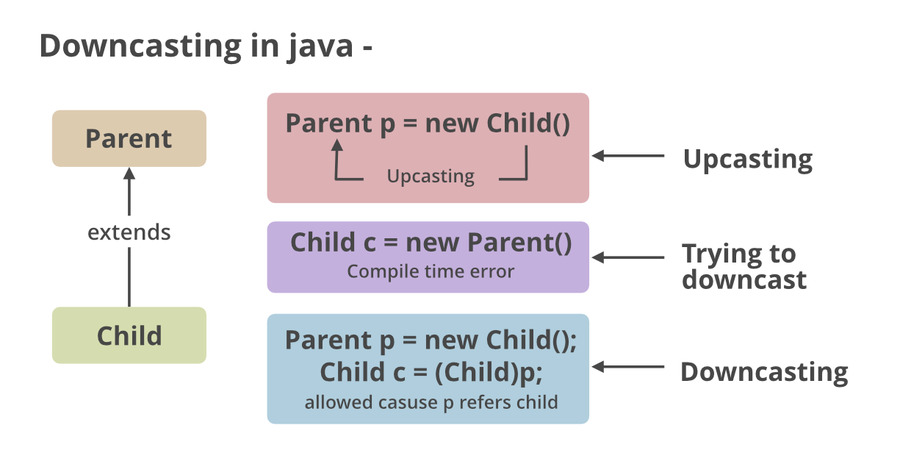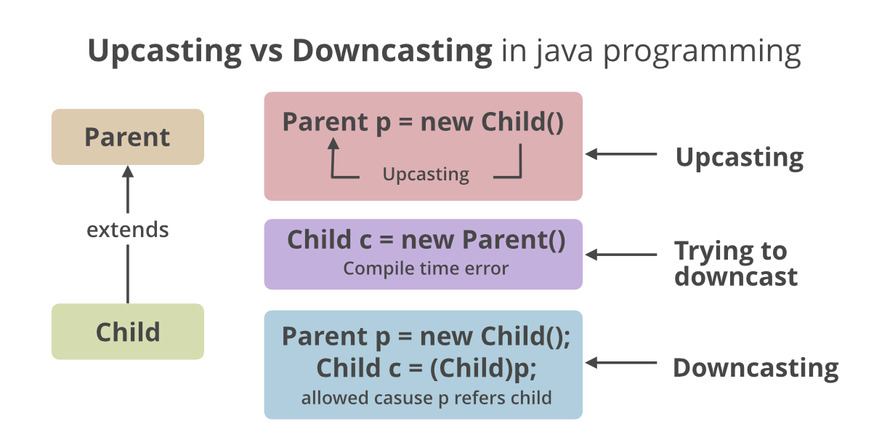# Class Type Casting in Java

• Difficulty Level : Expert
• Last Updated : 17 Sep, 2021

Typecasting is the assessment of the value of one primitive data type to another type. In java, there are two types of casting namely upcasting and downcasting as follows:

1. Upcasting is casting a subtype to a super type in an upward direction to the inheritance tree. It is an automatic procedure for which there are no efforts poured in to do so where a sub-class object is referred by a superclass reference variable. One can relate it with dynamic polymorphism.
• Implicit casting means class typecasting done by the compiler without cast syntax.
• Explicit casting means class typecasting done by the programmer with cast syntax.
2. Downcasting refers to the procedure when subclass type refers to the object of the parent class is known as downcasting. If it is performed directly compiler gives an error as ClassCastException is thrown at runtime. It is only achievable with the use of instanceof operator The object which is already upcast, that object only can be performed downcast.

In order to perform class type casting we have to follow these two rules as follows:

1. Classes must be “IS-A-Relationship “
2. An object must have the property of a class in which it is going to cast.

Implementation:

(A) Upcasting

Example 1

## Java

 `// Importing input output classes` `import` `java.io.*;`   `// Class 1` `// Parent class` `class` `Parent ` `{`   `  ``// Function ` `  ``void` `show()` `  ``{`   `    ``// Print message for this class` `    ``System.out.println(``"Parent show method is called"``);` `  ``}` `}  `   `// Class 2` `// Child class` `class` `Child ``extends` `Parent` `  ``{`   `    ``// Overriding existing method of Parent class` `    ``@Override`   `    ``// Same Function which will override ` `    ``// existing Parent class function` `    ``void` `show() ` `    ``{`   `    ``// Print message for this class` `    ``System.out.println(``"Child show method is called"``);` `    ``}`   `  ``}`   `// Class3` `// Main class` `class` `GFG ` `{`   `    ``// Main driver method` `    ``public` `static` `void` `main(String[] args)` `    ``{` `      ``// Creating a Parent class object ` `      ``// but referencing it to a Child class` `       ``Parent obj = ``new` `Child();` `      `  `      ``// Calling the show() method to execute` `       ``obj.show();` `    ``}` `}`

Output

`Child show method is called`

Output explanation: Here parent class object is called but referred to the child’s class object. Hence, one can relate this with dynamic polymorphism or function overriding.

(B) DowncastingExample 2

## Java

 `// Java Program to illustrate Downcasting`   `// Importing input output classes` `import` `java.io.*;`   `// Class 1` `// Parent class` `class` `Vehicles {` `}`   `// Class 2` `// Child class` `class` `Car ``extends` `Vehicles {` `    ``static` `void` `method(Vehicles v)` `    ``{`   `        ``//` `        ``if` `(v ``instanceof` `Car) {`   `            ``// Downcasting` `            ``Car c = (Car)v;`   `            ``// Display message` `            ``System.out.println(``"Downcasting performed"``);` `        ``}` `    ``}` `    ``// Main driver method` `    ``public` `static` `void` `main(String[] args)` `    ``{` `        ``// Creating an object of Vehicle class` `        ``// and referring it to Car class` `        ``Vehicles v = ``new` `Car();` `        ``Car.method(v);` `    ``}` `}`

Output

`Downcasting performed`

NOTE : Without perform upcast if we try to downcast , ClassCastException will be thrown.

• It is a runtime exception or unchecked exception.
• It is class, present in java.lang package.
• It can be avoided by using a operator known as ‘instanceof’.Example 3

## Java

 `// Java Program showing ClassCastException`   `// Importing input output classes` `import` `java.io.*;`   `// Class 1` `// Parent class/ Member class` `class` `Member {`   `    ``// Member variable of this class` `    ``String name;` `    ``long` `phone;`   `    ``// Member function of this class` `    ``void` `chat()` `    ``{`   `        ``// Print message of Member/ Child class` `        ``System.out.println(` `            ``name + ``" : chatting in whatsapp group"``);` `    ``}` `}`   `// Class 2` `// Child class/ Admin class` `class` `Admin ``extends` `Member {`   `    ``// Member function of this class` `    ``void` `addUser()` `    ``{`   `        ``// Print message of Admin/ Parent class` `        ``System.out.println(` `            ``name` `            ``+ ``" : adding a new user in whatsapp group"``);` `    ``}` `}`   `// Class3 - Main class` `class` `GFG {`   `    ``// Main driver method` `    ``public` `static` `void` `main(String[] args)` `    ``{`   `        ``// Creating an object Ad` `        ``Member mem = ``new` `Admin();`   `        ``// Upcasting access only general property of` `        ``// superclass`   `        ``// Custom entry for Member class` `        ``mem.name = ``"Sneha"``;` `        ``mem.phone = 9876543210l;` `        ``// Calling function` `        ``mem.chat();`   `        ``Admin ad = (Admin)mem;`   `        ``// Downcast to access specific property of subclass` `        ``ad.addUser();` `    ``}` `}`

Output

```Sneha : chatting in whatsapp group
Sneha : adding a new user in whatsapp group```

My Personal Notes arrow_drop_up
Recommended Articles
Page :